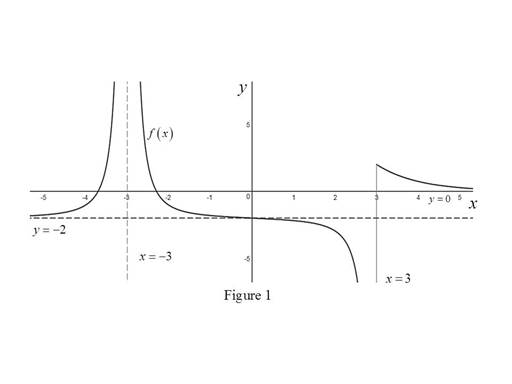# The graph of a function f.### Single Variable Calculus: Concepts...

4th Edition
James Stewart
Publisher: Cengage Learning
ISBN: 9781337687805### Single Variable Calculus: Concepts...

4th Edition
James Stewart
Publisher: Cengage Learning
ISBN: 9781337687805

#### Solutions

Chapter 2, Problem 2RE
To determine

## To sketch: The graph of a function f.

Expert Solution

### Explanation of Solution

Given:

The conditions are limxf(x)=2, limxf(x)=0, limx3f(x)=, limx3f(x)=, limx3+f(x)=2 and f is continuous from the right at 3.

Calculation:

Recall the definition of horizontal asymptote, “the line y=L is called a horizontal asymptote of the curve y=f(x) if limxf(x)=L or limxf(x)=L”.

Recall the definition that the line x=a is said to be a vertical asymptote of the function f(x), if at least one of the following conditions must be true: limxaf(x)=±, limxa+f(x)=±, limxaf(x)= and limxaf(x)=.

The limxf(x)=2 implies that y=2 is a horizontal asymptote to the function f.

The limxf(x)=0 implies that y=0 is a horizontal asymptote to the function f.

The limx3f(x)= implies that x=3 is a vertical asymptote to the function f.

The limx3f(x)= implies that x=3 is a vertical asymptote to the function f.

The limx3+f(x)=2 means that the limit of the function f(x) approaches 2 as x approaches 3 from the right.

Recall that a function f is continuous from the right at a number a if limxa+f(x)=f(a).

A function f is continuous from the right at a number 3 if limx3+f(x)=f(3).

Also limx3+f(x)=2. This implies that, f(3)=2.

Graph:

Use the above information and obtain the graph of f as shown below in Figure 1.Thus, the required graph of a function f.

### Have a homework question?

Subscribe to bartleby learn! Ask subject matter experts 30 homework questions each month. Plus, you’ll have access to millions of step-by-step textbook answers!